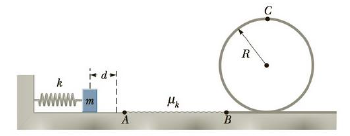Chapter 7, Problem 74AP

Chapter
Section
Textbook Problem

A massless spring of constant k = 78.4 N/m is fixed on the left side of a level track. A block of mass m = 0.50 kg is pressed against the spring and compresses it a distance d, as in Figure P7.74. The block (initially at rest) is then released and travels toward a circular loop-the-loop of radius R = 1.5 m. The entire track and the loop-the-loop are frictionless, except for the section of track between points A and B. Given that the coefficient of kinetic friction between the block and the track along AB is μk = 0.30 and that the length of AB is 2.5 m, determine the minimum compression d of the spring that enables the block to just make it through the loop-the-loop at point C. Hint: The force exerted by the track on the block will be zero if the block barely makes it through the loop-the-loop.Figure P7.74

To determine
The minimum compression of the spring.

Explanation

Given info: Mass of the block (m) is 0.50 kg. Length of the rod (L) is 1.50 m. The spring constant (k) is 78.4 N/m. The co-efficient of kinetic friction ( μk ) is 0.30. Length of AB is 2.5 m.

Explanation:

From the Work-Energy theorem,

W=12mv2+mg(2R)12kd2

• v is the speed of the block.
• R is the radius of the loop.
• g is the acceleration due to gravity.
• m is the mass of the block.

The work done is,

W=fklABcosθ

• fk is the force of friction.
• lAB is the length of AB.
• θ is the angle between the force and displacement.

The force of friction is,

fk=μkmg

The speed of the block is,

v=gR

Substitute the above equations in the Work-Energy theorem,

μkmglABcosθ=12m(gR)2+mg(2R)

Still sussing out bartleby?

Check out a sample textbook solution.

See a sample solution

The Solution to Your Study Problems

Bartleby provides explanations to thousands of textbook problems written by our experts, many with advanced degrees!

Get Started